GFG App
Open AppBrowser
Continue

# Class 8 RD Sharma Solutions – Chapter 8 Division Of Algebraic Expressions – Exercise 8.4 | Set 1

### Question 1. Divide 5x3 – 15x2 + 25x by 5x

Solution:

We have to divide 5x3 – 15x2 + 25x by 5x

So, (5x3 – 15x2 + 25x)/5x

5x3/5x – 15x2/5x + 25x/5x

x2 – 3x + 5

### Question 2. Divide 4z3 + 6z2 – z by −1/2 z

Solution:

We have to divide 4z3 + 6z2-z by −1/2 z

So, (4z3 + 6z2 – z) / (-1/2)z

(4z3/(-1/2)z) + (6z2/(-1/2)z) + (-z/(-1/2)z)

(-4 × 2/1)z2 + (6 × (-2)/1)z + (-1 × (-2/1))

-8z2 – 12z +2

### Question 3. Divide 9x2y – 6xy + 12xy2 by (−3/2) xy.

Solution:

We have to divide 9y – 6xy + 12xy² by (-3/2)xy

So, (9x2y – 6xy + 12xy2) / (-3/2)xy

(9x2y/(-3/2)xy ) – (6xy/(-3/2)xy) + (12xy2/(-3/2)xy)

(9 × (-2/3)x) – (6 × (-2/3)) + (12 × (-2/3))y

-6x -8y +4

### Question 4. Divide 3x2y2 + 2x2y + 15xy by 3xy

Solution:

We have to divide 3x2y2 + 2x2y + 15xy by 3xy

So, (x2y2 + 2x2y + 15xy) / 3xy

(3x2y2/3xy) + (2x2y/3xy) + (15xy/3xy)

x2y + (2/3)x +5

### Question 5. Divide x2 + 7x + 12 by x + 4

Solution:

We have to divide x2 + 7x + 12 by x + 4

So by using long division method we get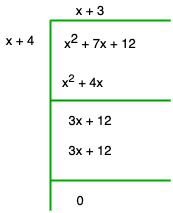Quotient = x + 3

Remainder = 0

### Question 6. Divide 4y2 + 3y + 1/2 by 2y + 1

Solution:

We have to divide 4y2 + 3y + 12 by 2y + 1

So by using long division method we get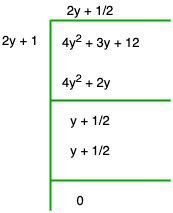Quotient = 2y + 1/2

Remainder = 0

### Question 7. Divide 3x3 + 4x2 + 5x + 18 by x + 2

Solution:

We have to divide 3x3 + 4x2 + 5x + 18 by x + 2

-2x2 – 4x + 18

So by using long division method we get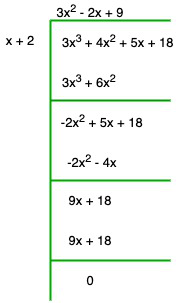Quotient = 3x2 – 2x + 9

Remainder = 0

### Question 8. Divide 14x2 – 53x + 45 by 7x – 9

Solution:

We have to divide 14x2 – 53x + 45 by 7x – 9

So by using long division method we get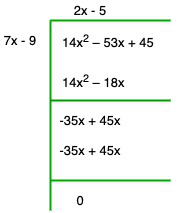Quotient = 2x – 5

Remainder = 0

### Question 9. Divide -21 + 71x – 31x2 – 24x3 by 3 – 8x

Solution:

We have to divide -21 + 71x – 31x2 – 24x3 by 3 – 8x

So by using long division method we get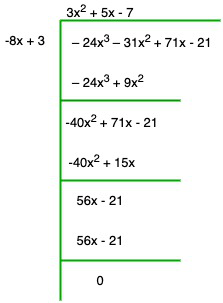Quotient = 3x2 + 5x – 7

Remainder = 0

### Question 10. Divide 3y4 – 3y3 – 4y2 – 4y by y2 – 2y

Solution:

We have to divide 3y4 – 3y3 – 4y2 – 4y by y2 – 2y

2y2 – 4y

So by using long division method we get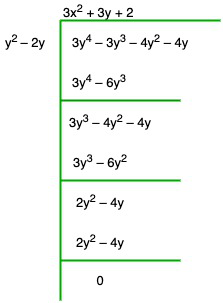Quotient = 3x2 + 3y + 2

Remainder = 0

### Question 11. Divide 2y5 + 10y4 + 6y3 + y2 + 5y + 3 by 2y3 + 1

Solution:

We have to divide 2y5 + 10y4 + 6y3 + y2 + 5y + 3 by 2y3 + 1

So by using long division method we get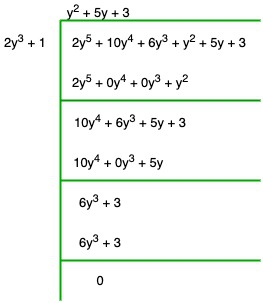Quotient = y2 + 5y + 3

Remainder = 0

### Question 12. Divide x4 – 2x3 + 2x2 + x + 4 by x2 + x + 1.

Solution:

We have to divide x4 – 2x3 + 2x2 + x + 4 by x2 + x + 1

4x2 + 4x + 4

So by using long division method we get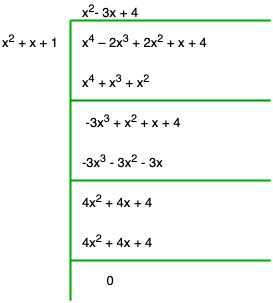Quotient = x2– 3x + 4

Remainder = 0

### Question 13. Divide m3 – 14m2 + 37m – 26 by m2 – 12m + 13.

Solution:

We have to divide m3 – 14m2 + 37m – 26 by m2 – 12m + 13

-2m2 + 24m – 26

So by using long division method we get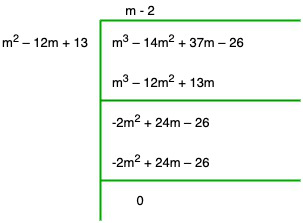Quotient = m – 2

Remainder = 0

### Question 14. Divide x4 + x2 + 1 by x2 + x + 1

Solution:

We have to divide x4 + x2 + 1 by x2 + x + 1

So by using long division method we get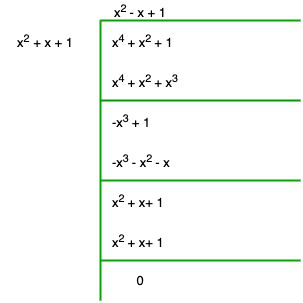Quotient = x2 – x + 1

Remainder = 0

### Question 15. Divide x5 + x4 + x3 + x2 + x + 1 by x3 + 1

Solution:

We have to divide x5 + x4 + x3 + x2 + x + 1 by x3 + 1

x3 + 1

So by using long division method we get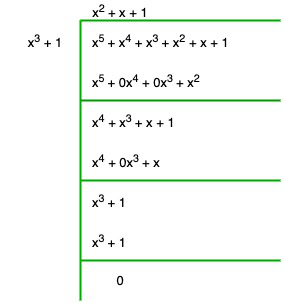Quotient = x2 + x + 1

Remainder = 0

### Question 16. Divide 14x3 – 5x2 + 9x – 1 by 2x – 1

Solution:

We have to divide 14x3 – 5x2 + 9x – 1 by 2x – 1

So by using long division method we get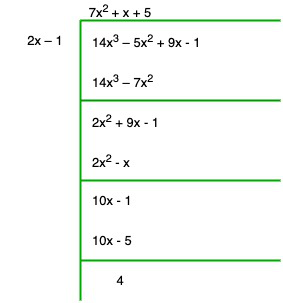Quotient = 7x2 + x + 5

Remainder = 4

### Question 17. Divide 6x3 – x2 – 10x – 3 by 2x – 3

Solution:

We have to divide 6x3 – x2 – 10x – 3 by 2x – 3

So by using long division method we get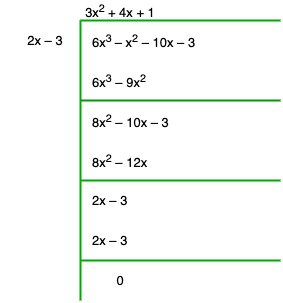Quotient = 3x2 + 4x + 1

Remainder = 0

### Question 18. Divide 6x3 + 11x2 – 39x – 65 by 3x2 + 13x + 13

Solution:

We have to divide 6x3 + 11x2 – 39x – 65 by 3x2 + 13x + 13

So by using long division method we get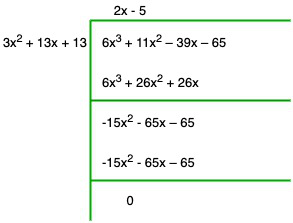Quotient = 2x – 5

Remainder = 0

My Personal Notes arrow_drop_up